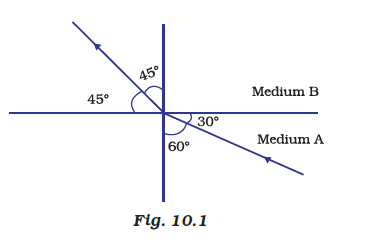# Figure shows a ray of light as it travels from medium A to medium B. Refractive index of the medium B relative to medium A is (a) Root 3 / Root 2 (b) 2 / 3 (c) 1/ 2 (d) 2Refractive Index of B with respect to A

The ratio of the sine of the angle of incidence to the sine of the angle of refraction is a constant, for the light of a given colour and for the given pair of media. This law is also known as Snell’s law of refraction of light. The law follows from the boundary condition that a wave is continuous across a boundary, which requires that the phase of the wave be constant on any given plane.

n = sin i/sin r

n = sin 60/sin 45

n = (√3 /2) /(1/√2)

n = √3/√2(8)(15)